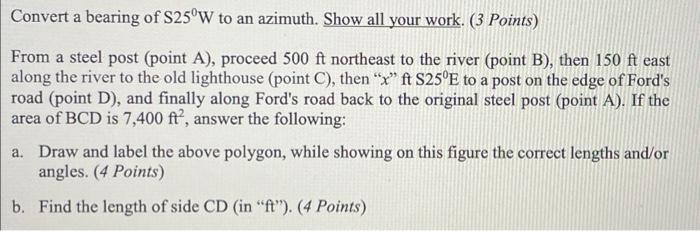Home / Expert Answers / Civil Engineering / convert-a-bearing-of-mathrm-s-25-circ-mathrm-w-to-an-azimuth-show-all-your-work-3-pa703

# (Solved): Convert a bearing of $$\mathrm{S} 25^{\circ} \mathrm{W}$$ to an azimuth. Show all your work. ( 3 ...Convert a bearing of $$\mathrm{S} 25^{\circ} \mathrm{W}$$ to an azimuth. Show all your work. ( 3 Points) From a steel post (point A), proceed $$500 \mathrm{ft}$$ northeast to the river (point B), then $$150 \mathrm{ft}$$ east along the river to the old lighthouse (point C), then " $$x$$ " $$\mathrm{ft} \mathrm{S} 25^{\circ} \mathrm{E}$$ to a post on the edge of Ford's road (point D), and finally along Ford's road back to the original steel post (point A). If the area of $$\mathrm{BCD}$$ is $$7,400 \mathrm{ft}^{2}$$, answer the following: a. Draw and label the above polygon, while showing on this figure the correct lengths and/or angles. (4 Points) b. Find the length of side $$\mathrm{CD}$$ (in " $$\mathrm{ft}$$ "). (4 Points)

We have an Answer from Expert